# Chapter 14 The chordDiagram() function

One unique feature of circular layout is the circular visualization of relations between objects by links. See examples in http://circos.ca/intro/tabular_visualization/. Such type of plot is called Chord diagram. In circlize, it is easy to plot Chord diagram in a straightforward or in a highly customized way.

There are two data formats that represent relations, either adjacency matrix or adjacency list. In adjacency matrix, value in $$i^{th}$$ row and $$j^{th}$$ column represents the relation from object in the $$i^{th}$$ row and the object in the $$j^{th}$$ column where the absolute value measures the strength of the relation. In adjacency list, relations are represented as a three-column data frame in which relations come from the first column and to the second column, and the third column represents the strength of the relation.

Following code shows an example of an adjacency matrix.

mat = matrix(1:9, 3)
rownames(mat) = letters[1:3]
colnames(mat) = LETTERS[1:3]
mat
##   A B C
## a 1 4 7
## b 2 5 8
## c 3 6 9

And the code in below is an example of an adjacency list.

df = data.frame(from = letters[1:3], to = LETTERS[1:3], value = 1:3)
df
##   from to value
## 1    a  A     1
## 2    b  B     2
## 3    c  C     3

Actually, it is not difficult to convert between these two formats. There are also R packages and functions do the conversion such as in reshape2 package, melt() converts a matrix to a data frame and dcast() converts the data frame back to the matrix.

Chord diagram shows the information of the relation from several levels. 1. the links are straightforward to show the relations between objects; 2. width of links are proportional to the strength of the relation which is more illustrative than other graphic mappings; 3. colors of links can be another graphic mapping for relations; 4. width of sectors represents total strength for an object which connects to other objects or is connected from other objects. You can find an interesting example of using Chord diagram to visualize leagues system of players clubs by their national team from https://gjabel.wordpress.com/2014/06/05/world-cup-players-representation-by-league-system/ and the adapted code is at https://jokergoo.github.io/circlize_examples/example/wc2014.html.

In circlize package, there is a chordDiagram() function that supports both adjacency matrix and adjacency list. For different formats of input, the corresponding format of graphic parameters will be different either. E.g. when the input is a matrix, since information of the links in the Chord diagram is stored in the matrix, corresponding graphics for the links sometimes should also be specified as a matrix, while if the input is a data frame, the graphic parameters for links only need to be specified as an additional column to the data frame. Using the matrix is very straightforward and makes goodlooking plots, while using the data frame provides more flexibility for controlling the Chord diagram. Thus, in this chapter, we will show usage for both adjacency matrix and list.

Since the usage for the two types of inputs are highly similar, in this chapter, we mainly show figures generated from the matrix, but still show the code which uses adjacency list.

## 14.1 Basic usage of making Chord diagram

First let’s generate a random matrix and the corresponding adjacency list.

set.seed(999)
mat = matrix(sample(18, 18), 3, 6)
rownames(mat) = paste0("S", 1:3)
colnames(mat) = paste0("E", 1:6)
mat
##    E1 E2 E3 E4 E5 E6
## S1  4 14 13 17  5  2
## S2  7  1  6  8 12 15
## S3  9 10  3 16 11 18
df = data.frame(from = rep(rownames(mat), times = ncol(mat)),
to = rep(colnames(mat), each = nrow(mat)),
value = as.vector(mat),
stringsAsFactors = FALSE)
df
##    from to value
## 1    S1 E1     4
## 2    S2 E1     7
## 3    S3 E1     9
## 4    S1 E2    14
## 5    S2 E2     1
## 6    S3 E2    10
## 7    S1 E3    13
## 8    S2 E3     6
## 9    S3 E3     3
## 10   S1 E4    17
## 11   S2 E4     8
## 12   S3 E4    16
## 13   S1 E5     5
## 14   S2 E5    12
## 15   S3 E5    11
## 16   S1 E6     2
## 17   S2 E6    15
## 18   S3 E6    18

The simplest usage is just calling chordDiagram() with mat (Figure 14.1).

chordDiagram(mat)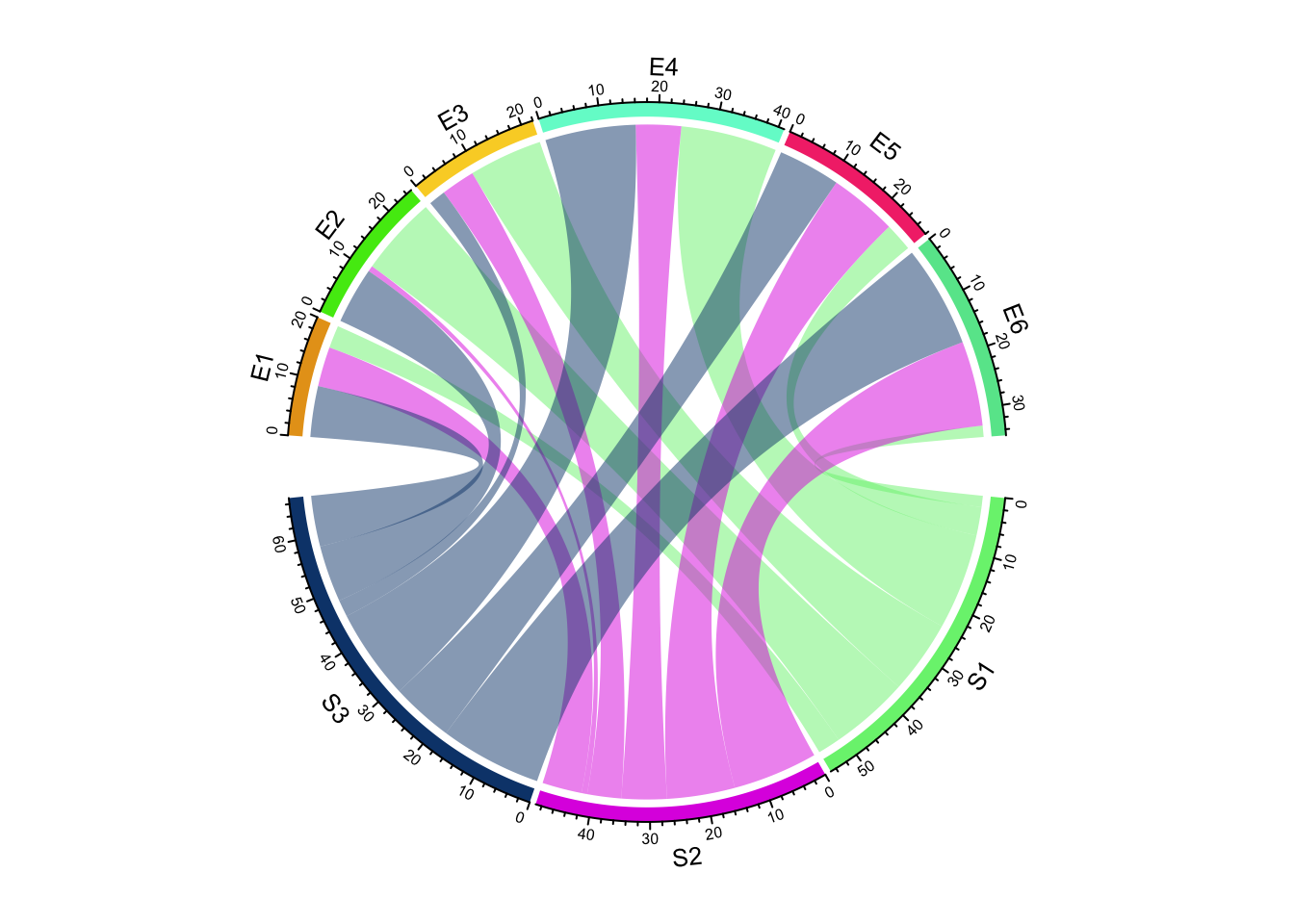Figure 14.1: Basic usages of chordDiagram().

circos.clear()

or call with df:

chordDiagram(df)
circos.clear()

The default Chord Diagram consists of a track of labels, a track of grids (or you can call it lines, rectangles) with axes, and links. Sectors which correspond to rows in the matrix locate at the bottom half of the circle. The order of sectors is the order of union(rownames(mat), colnames(mat)) or union(df[], df[]) if input is a data frame. The order of sectors can be controlled by order argument (Figure 14.2, left). Of course, the length of order vector should be same as the number of sectors.

In following code, S1, S2 and S3 should better be put together since they belong to a same group, which is same for E* sectors. Of course, you can give a order which mix S* and E* (Figure 14.2, right), but it is not recommended because it is bad for reading the plot. You can find more information of setting grouped Chord diagrams (two or more groups) in Section 15.6.

par(mfrow = c(1, 2))
chordDiagram(mat, order = c("S2", "S1", "S3", "E4", "E1", "E5", "E2", "E6", "E3"))
circos.clear()

chordDiagram(mat, order = c("S2", "S1", "E4", "E1", "S3", "E5", "E2", "E6", "E3"))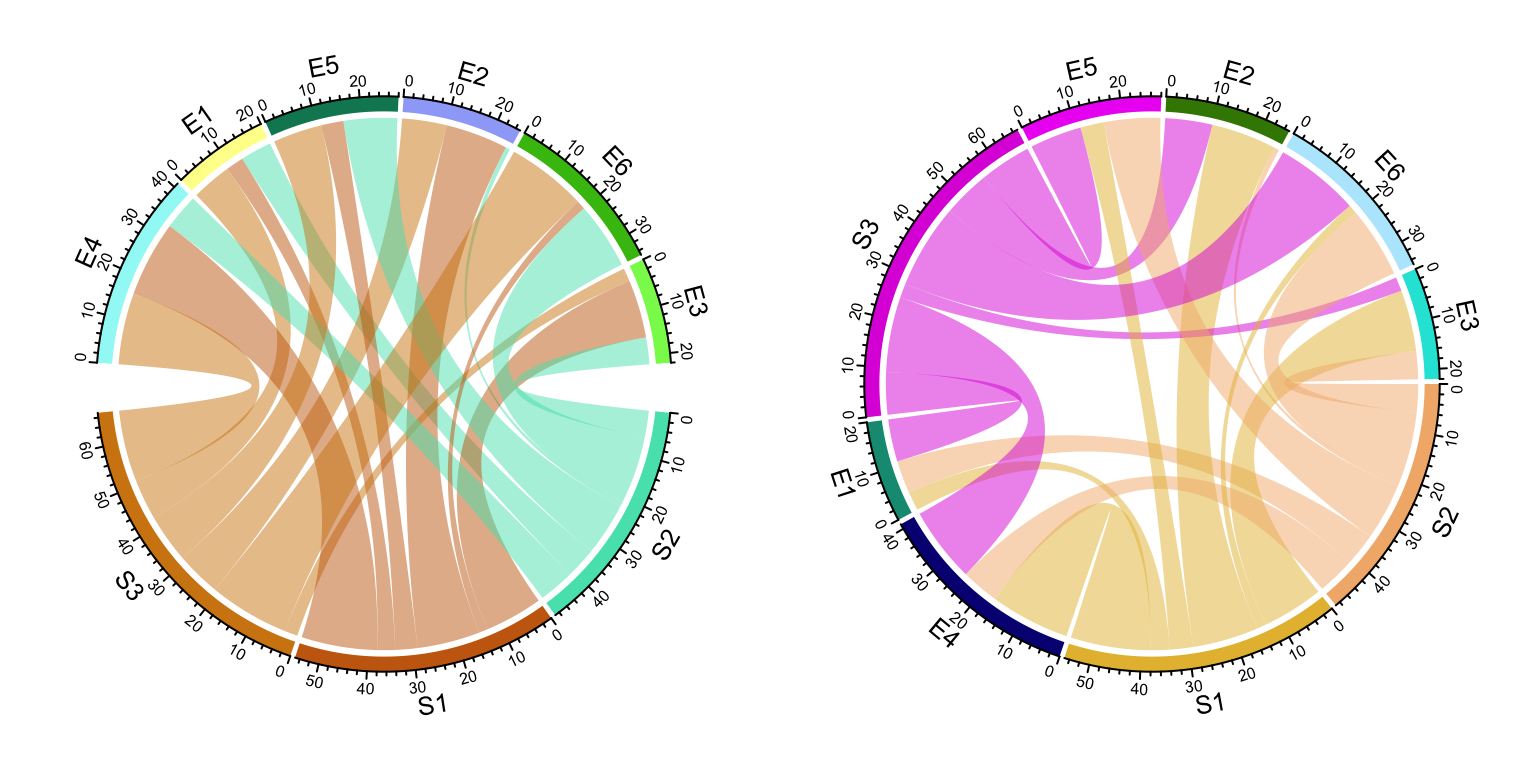Figure 14.2: Adjust sector orders in Chord diagram. Left: sectors are still grouped after reordering; right: sectors are not grouped.

circos.clear()

Under default settings, the grid colors which represent sectors are randomly generated, and the link colors are same as grid colors which correspond to rows (or the first column if the input is an adjacency list) but with 50% transparency.

## 14.2 Adjust by circos.par()

Since Chord Diagram is implemented by basic circlize functions, like normal circular plot, the layout can be customized by circos.par().

The gaps between sectors can be set by circos.par(gap.after = ...) (Figure 14.3). It is useful when you want to distinguish sectors between rows and columns. Please note since you change default graphical settings, you need to use circos.clear() in the end of the plot to reset it.

circos.par(gap.after = c(rep(5, nrow(mat)-1), 15, rep(5, ncol(mat)-1), 15))
chordDiagram(mat)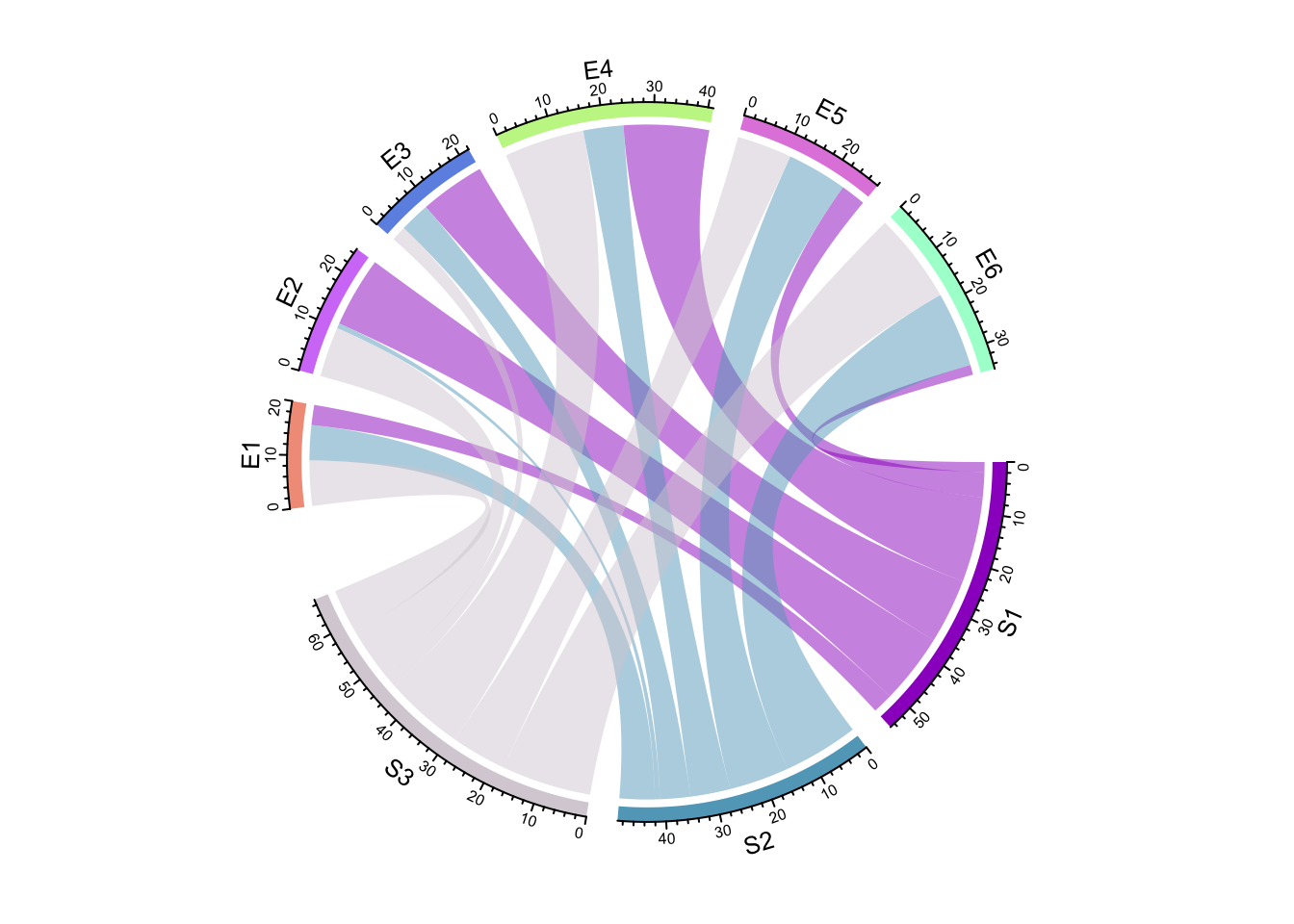Figure 14.3: Set gaps in Chord diagram.

circos.clear()

If the input is a data frame:

circos.par(gap.after = c(rep(5, length(unique(df[]))-1), 15,
rep(5, length(unique(df[]))-1), 15))
chordDiagram(df)
circos.clear()

As explained in Section 2.4, gap.after can also specified as a named vector:

circos.par(gap.after = c("S1" = 5, "S2" = 5, "S3" = 15, "E1" = 5, "E2" = 5,
"E3" = 5, "E4" = 5, "E5" = 5, "E6" = 15))
chordDiagram(mat)
circos.clear()

To make it simpler, users can directly set big.gap in chordDiagram() (Figure 14.4). The value of big.gap corresponds to the gap between row sectors and column sectors (or first-column sectors and second-column sectors in the input is a data frame). Internally a proper gap.after is assigned to circos.par(). Note it will only work when there is no overlap between row sectors and column sectors, or in other words, rows and columns in the matrix, or the first column and the second column in the data frame represent two different groups.

chordDiagram(mat, big.gap = 30)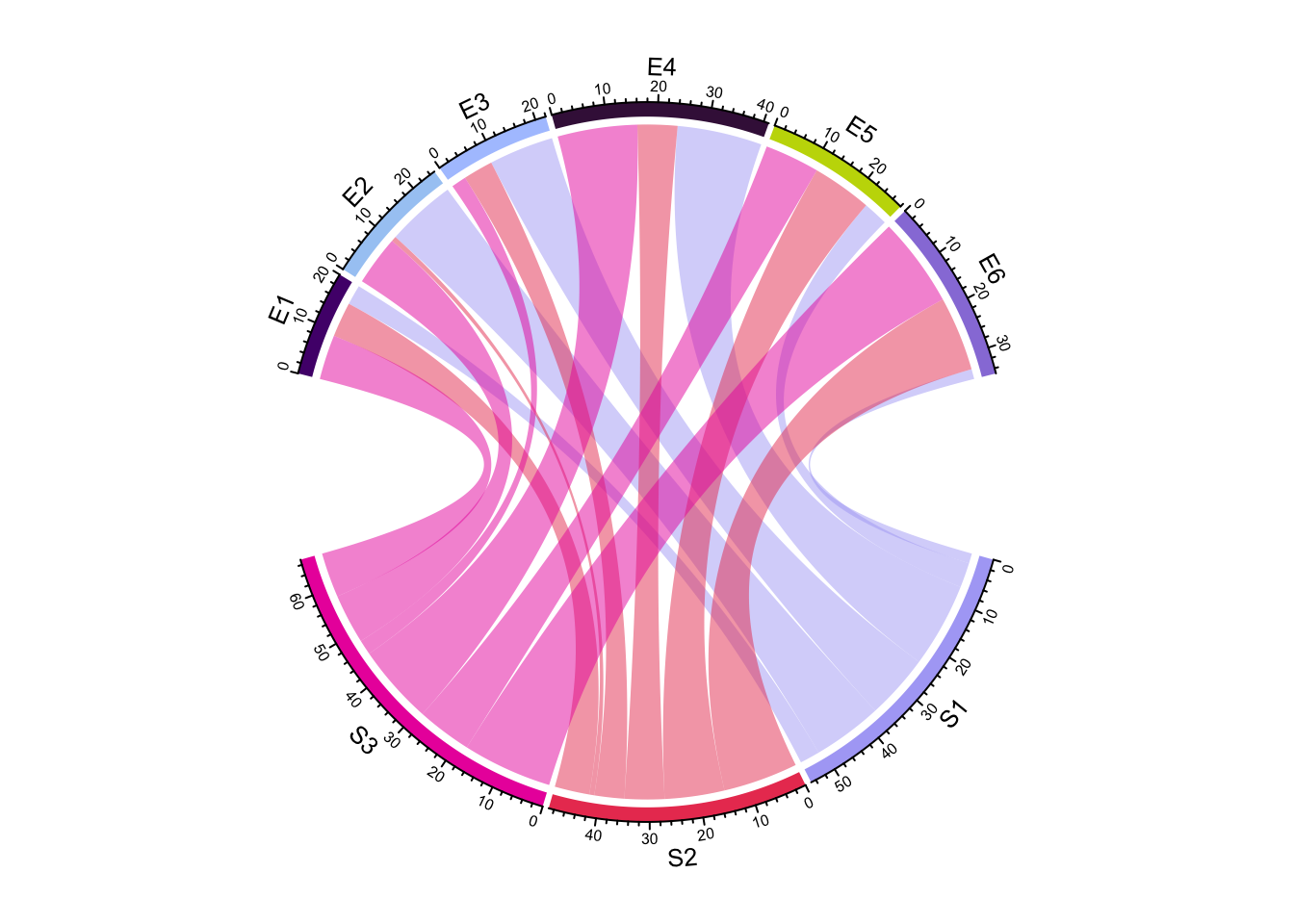Figure 14.4: Directly set big.gap in chordDiagram().

circos.clear()

small.gap argument controls the gap between sectors corresponding to either matrix rows or columns. The default value is 1 degree and normally you don’t need to set it. big.gap and small.gap can also be set in the scenarios where number of groups is larger than two, see Section 15.6 for more details.

Similar to a normal circular plot, the first sector (which is the first row in the adjacency matrix or the first row in the adjacency list) starts from right center of the circle and sectors are arranged clock-wisely. The start degree for the first sector can be set by circos.par(start.degree = ...) and the direction can be set by circos.par(clock.wise = ...) (Figure 14.5).

In Figure 14.5 (left), setting circos.par(clock.wise = FALSE) makes the link so twisted. Actually making the direction reverse clock wise can also be done by setting a reversed order of all sectors (Figure 14.5, right). As we can see, the links in the left plot are very twisted, while it still looks fine in the right plot. The reason is chordDiagram() automatically optimizes the positions of links according to the arrangement of sectors.

par(mfrow = c(1, 2))
circos.par(start.degree = 85, clock.wise = FALSE)
chordDiagram(mat)
circos.clear()

circos.par(start.degree = 85)
chordDiagram(mat, order = c(rev(colnames(mat)), rev(rownames(mat))))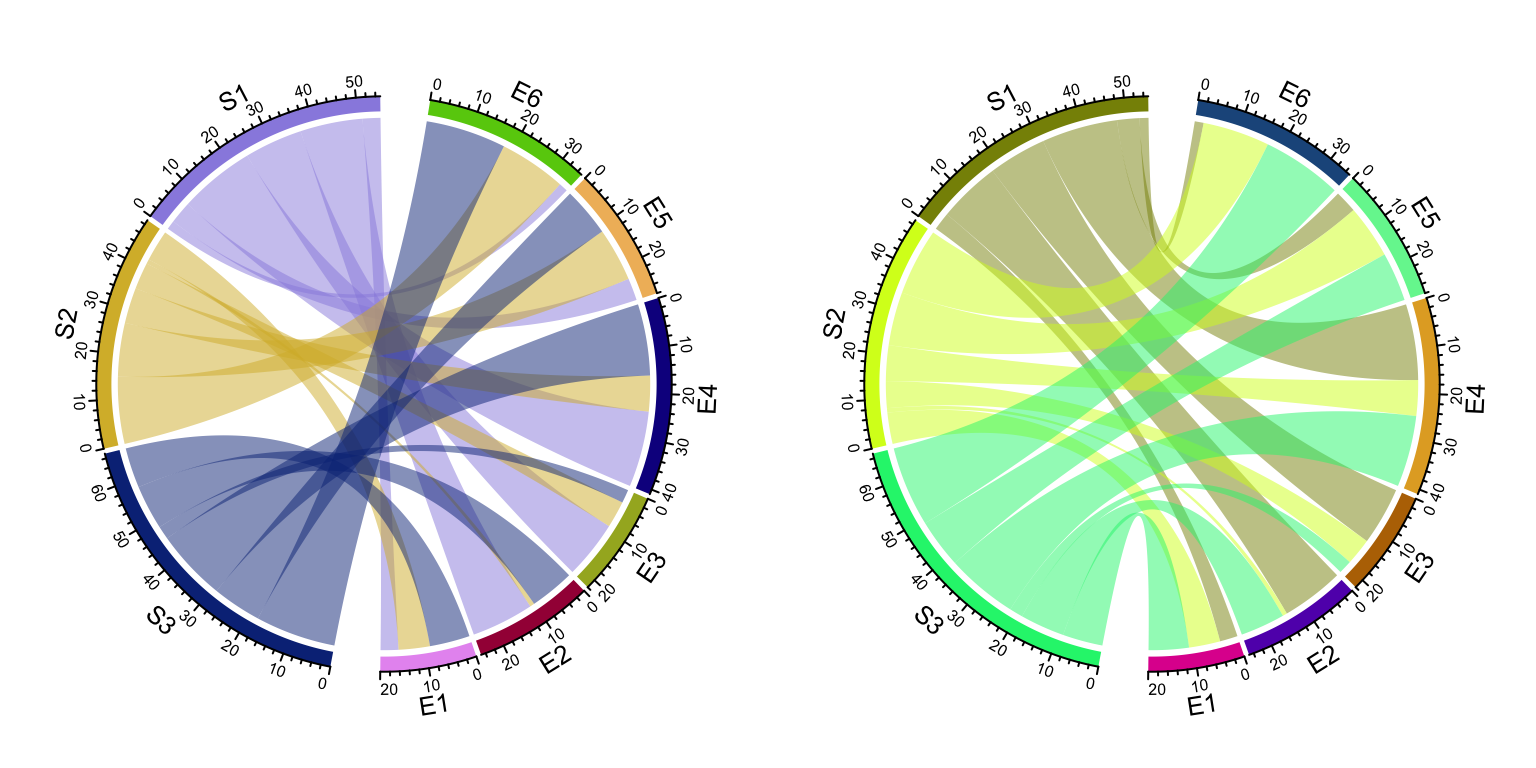Figure 14.5: Change position and orientation of Chord diagram.

circos.clear()

## 14.3 Colors

### 14.3.1 Set grid colors

Grids have different colors to represent different sectors. Generally, sectors are divided into two groups. One contains sectors defined in rows of the matrix or the first column in the data frame, and the other contains sectors defined in columns of the matrix or the second column in the data frame. Thus, links connect objects in the two groups. By default, link colors are same as the color for the corresponding sectors in the first group.

Changing colors of grids may change the colors of links as well. Colors for grids can be set through grid.col argument. Values of grid.col better be a named vector of which names correspond to sector names. If it is has no name index, the order of grid.col is assumed to have the same order as sectors (Figure 14.6). If you want to colors to be the same as the sectors from the matrix columns or the second column in the data frame, simply transpose the matrix (Figure 14.6 right).

grid.col = c(S1 = "red", S2 = "green", S3 = "blue",
E1 = "grey", E2 = "grey", E3 = "grey", E4 = "grey", E5 = "grey", E6 = "grey")
chordDiagram(mat, grid.col = grid.col)
chordDiagram(t(mat), grid.col = grid.col)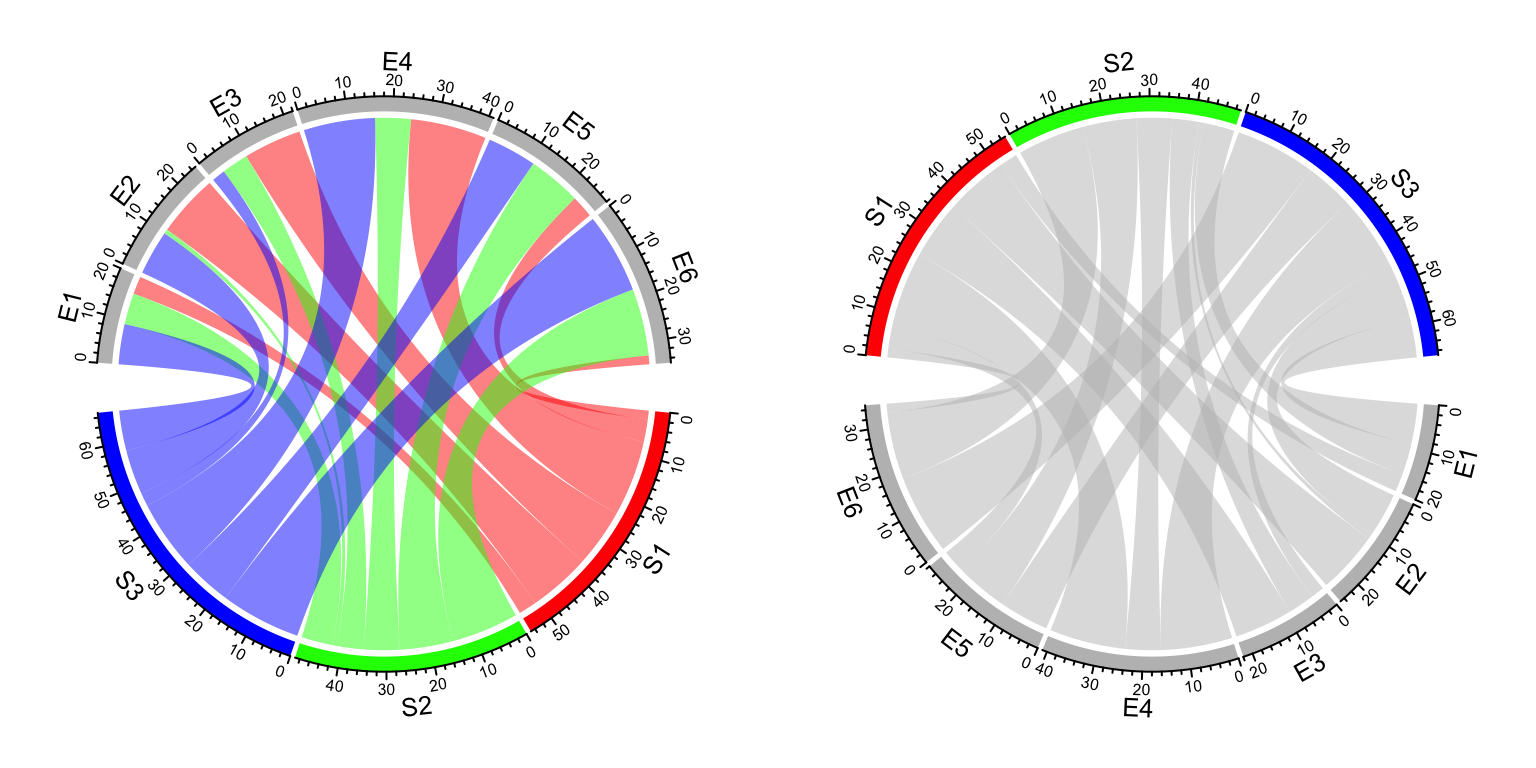Figure 14.6: Set grid colors in Chord diagram.

## 14.9 Symmetric matrix

When the matrix is symmetric, by setting symmetric = TRUE, only lower triangular matrix without the diagonal will be used (Figure 14.22).

mat3 = matrix(rnorm(25), 5)
colnames(mat3) = letters[1:5]
cor_mat = cor(mat3)
col_fun = colorRamp2(c(-1, 0, 1), c("green", "white", "red"))
chordDiagram(cor_mat, grid.col = 1:5, symmetric = TRUE, col = col_fun)
title("symmetric = TRUE")
chordDiagram(cor_mat, grid.col = 1:5, col = col_fun)
title("symmetric = FALSE")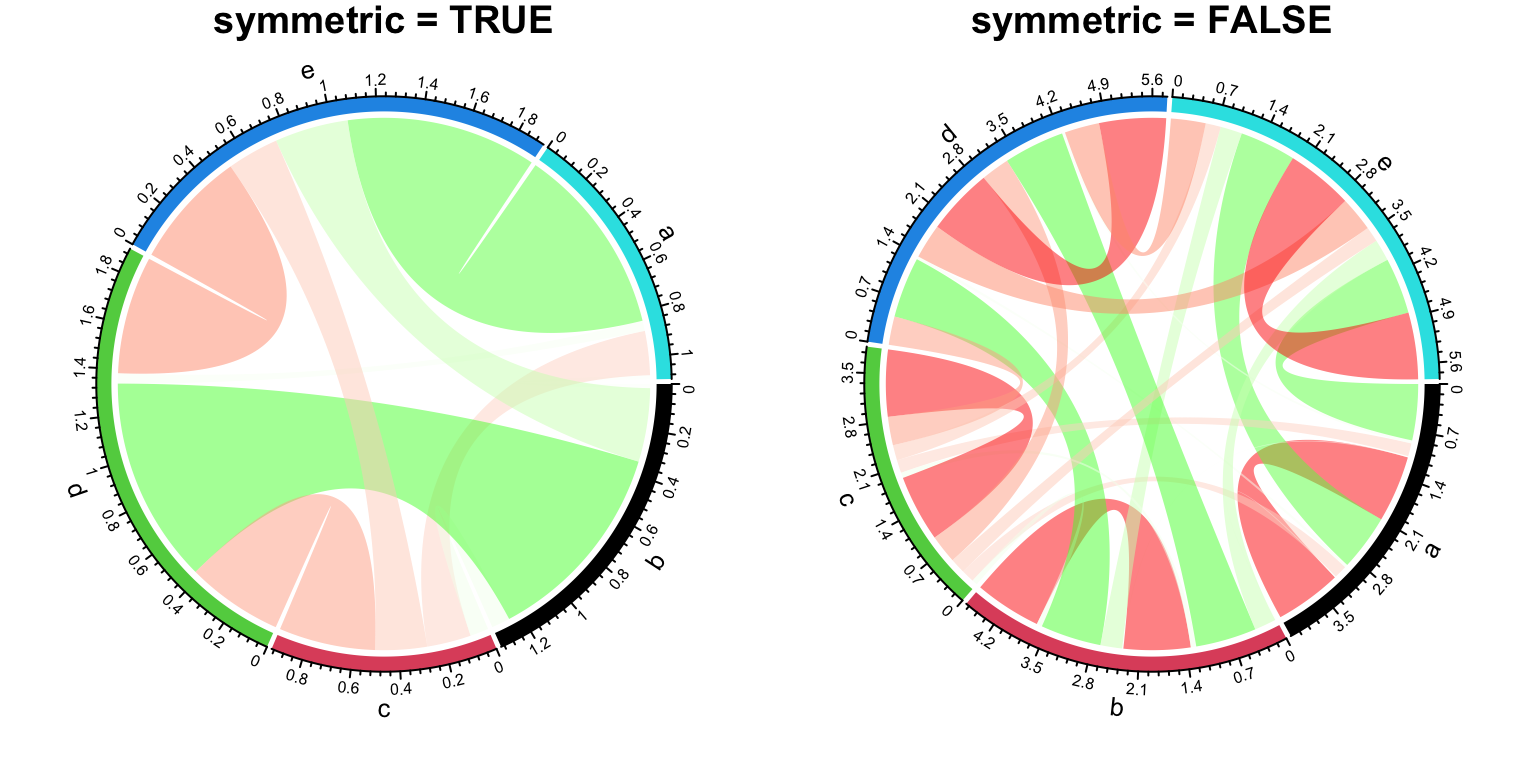Figure 14.22: Symmetric matrix for Chord diagram.

## 14.10 Directional relations

In some cases, when the input is a matrix, rows and columns represent directions, or when the input is a data frame, the first column and second column represent directions. Argument directional is used to illustrate such direction on the plot. directional with value 1 means the direction is from rows to columns (or from the first column to the second column for the adjacency list) while -1 means the direction is from columns to rows (or from the second column to the first column for the adjacency list). A value of 2 means bi-directional.

By default, the two ends of links have unequal height (Figure 14.23) to represent the directions. The position of starting end of the link is shorter than the other end to give users the feeling that the link is moving out. If this is not what your correct feeling, you can set diffHeight to a negative value.

par(mfrow = c(1, 3))
chordDiagram(mat, grid.col = grid.col, directional = 1)
chordDiagram(mat, grid.col = grid.col, directional = 1, diffHeight = mm_h(5))
chordDiagram(mat, grid.col = grid.col, directional = -1)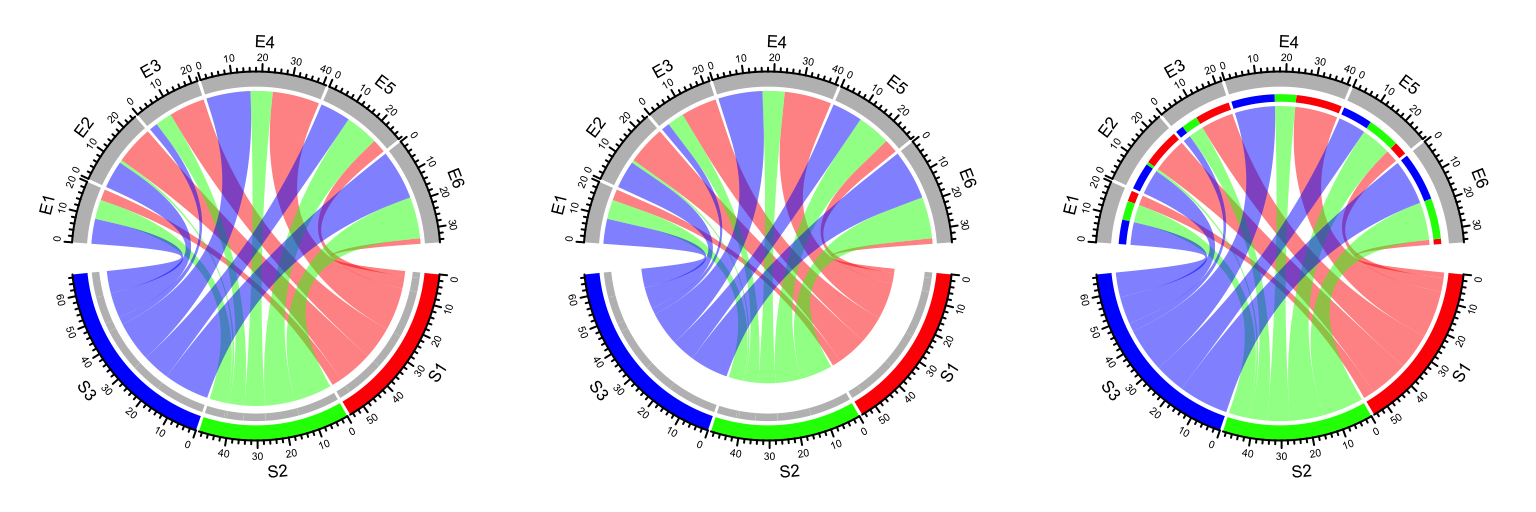Figure 14.23: Represent directions by different height of link ends.

Row names and column names in mat can also overlap. In this case, showing direction of the link is important to distinguish them (Figure 14.24).

mat2 = matrix(sample(100, 35), nrow = 5)
rownames(mat2) = letters[1:5]
colnames(mat2) = letters[1:7]
mat2
##    a  b  c  d  e  f  g
## a 60 14 33 27 70  2 45
## b 89 84 87  9 78 49 40
## c 26  7 42 74 36 76  4
## d 51 82 85 16 73 59 31
## e 30 90 23 38 68 11 35
chordDiagram(mat2, grid.col = 1:7, directional = 1, row.col = 1:5)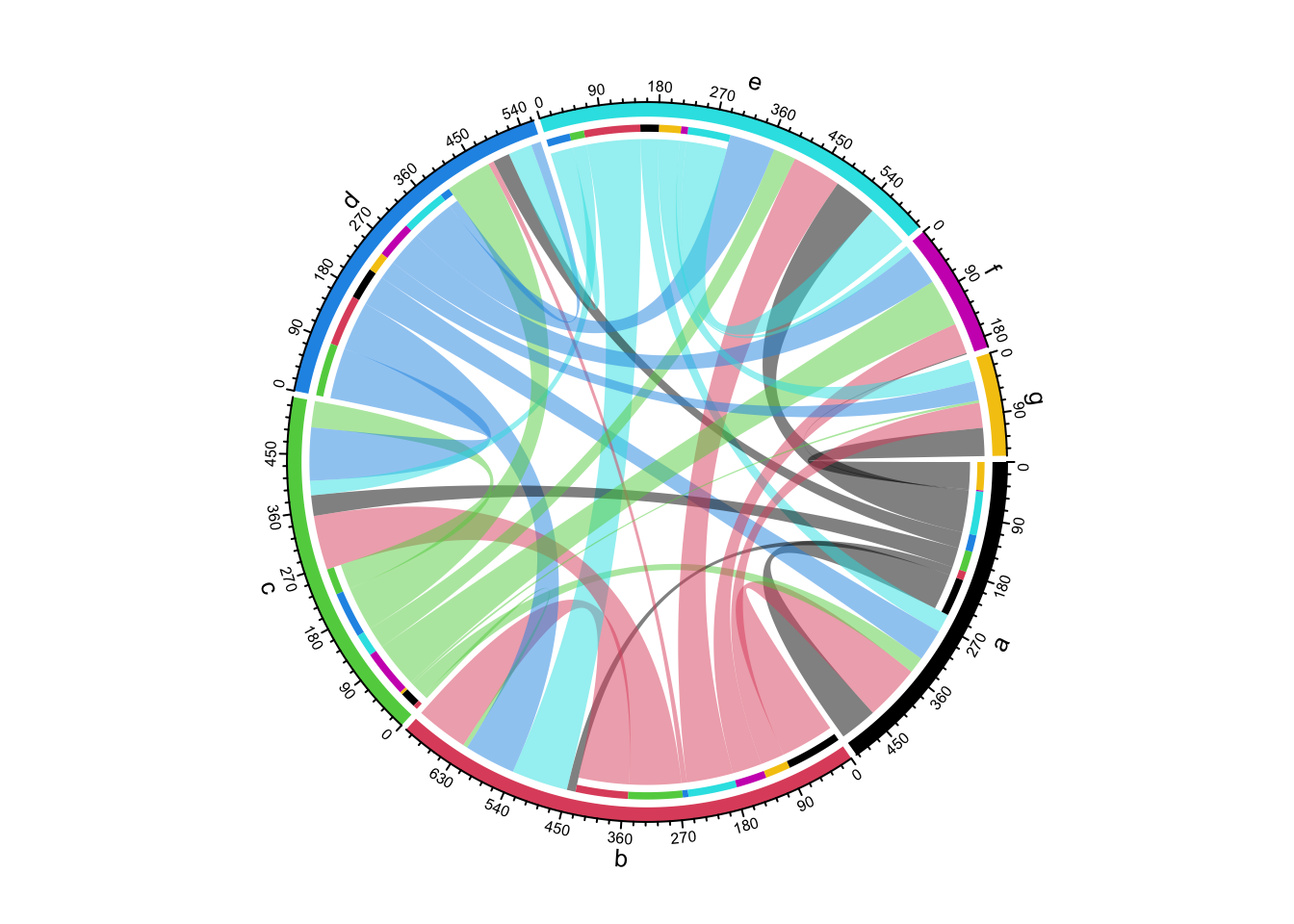Figure 14.24: Chord diagram where row names and column names overlap.

If you do not need self-link for which two ends of a link are in a same sector, just set corresponding values to 0 in the matrix (Figure 14.25).

mat3 = mat2
for(cn in intersect(rownames(mat3), colnames(mat3))) {
mat3[cn, cn] = 0
}
mat3
##    a  b  c  d  e  f  g
## a  0 14 33 27 70  2 45
## b 89  0 87  9 78 49 40
## c 26  7  0 74 36 76  4
## d 51 82 85  0 73 59 31
## e 30 90 23 38  0 11 35
chordDiagram(mat3, grid.col = 1:7, directional = 1, row.col = 1:5)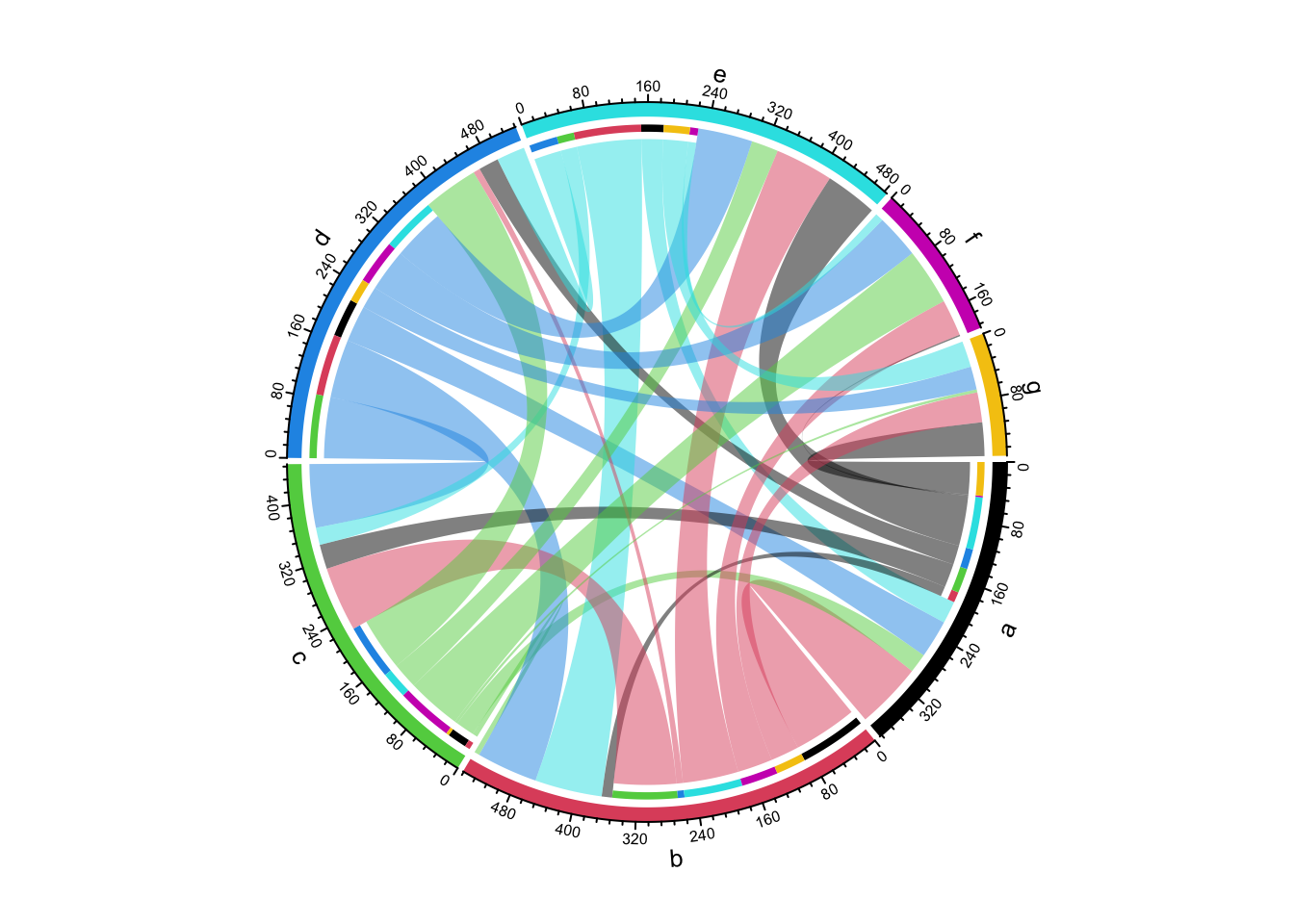Figure 14.25: Directional Chord diagram without self links.

Links can have arrows to represent directions (Figure 14.26). When direction.type is set to arrows, Arrows are added at the center of links. Similar as other graphics parameters for links, the parameters for drawing arrows such as arrow color and line type can either be a scalar, a matrix, or a three-column data frame.

If link.arr.col is set as a data frame, only links specified in the data frame will have arrows. Pleast note this is the only way to draw arrows to subset of links.

arr.col = data.frame(c("S1", "S2", "S3"), c("E5", "E6", "E4"),
c("black", "black", "black"))
chordDiagram(mat, grid.col = grid.col, directional = 1, direction.type = "arrows",
link.arr.col = arr.col, link.arr.length = 0.2)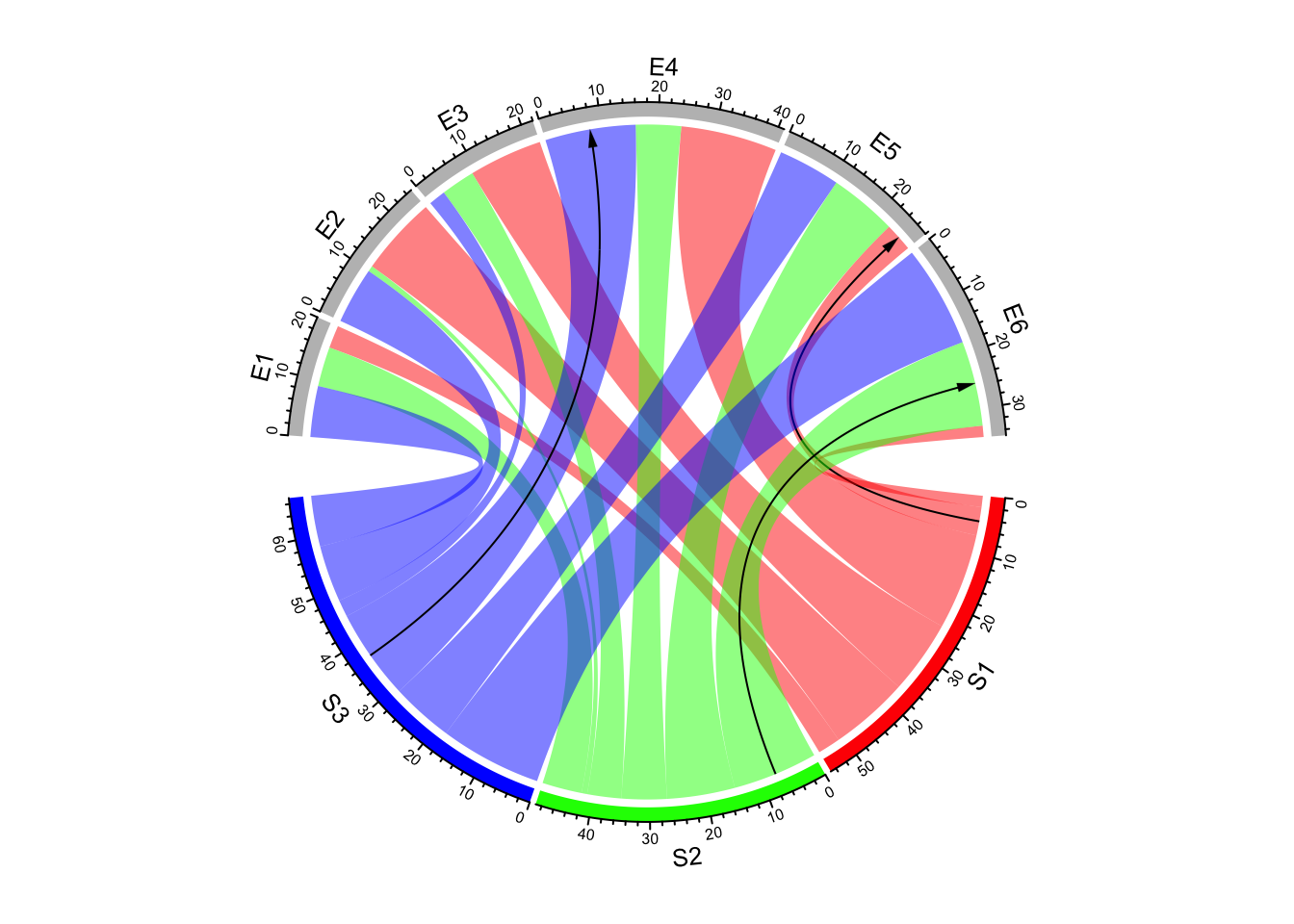Figure 14.26: Use arrows to represent directions in Chord diagram.

If combining both arrows and diffHeight, it will give you better visualization (Figure 14.27).

arr.col = data.frame(c("S1", "S2", "S3"), c("E5", "E6", "E4"),
c("black", "black", "black"))
chordDiagram(mat, grid.col = grid.col, directional = 1,
direction.type = c("diffHeight", "arrows"),
link.arr.col = arr.col, link.arr.length = 0.2)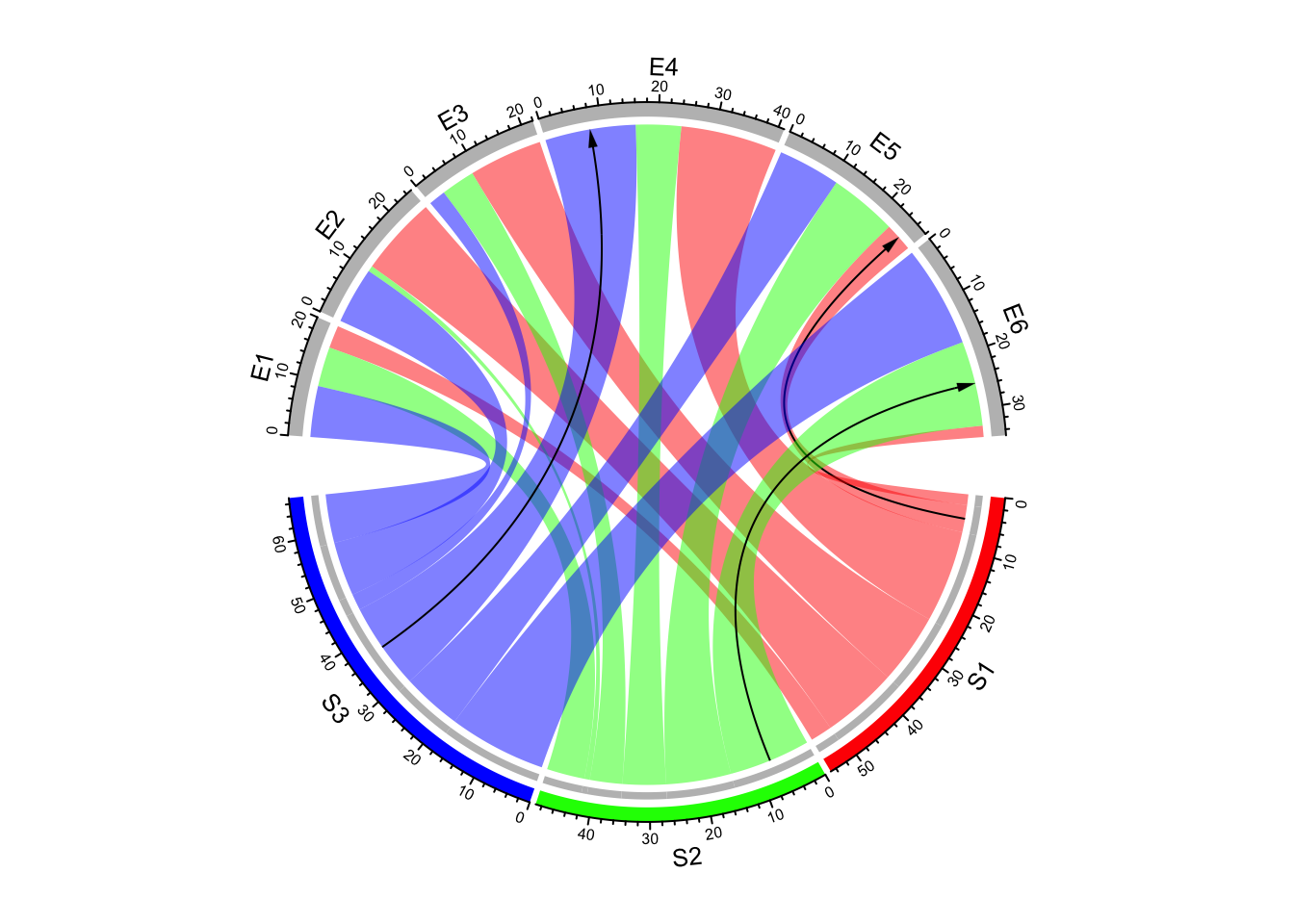Figure 14.27: Use both arrows and link height to represent directions in Chord diagram.

There is another arrow type: big.arrow which is efficient to visualize arrows when there are too many links (Figure 14.28).

matx = matrix(rnorm(64), 8)
chordDiagram(matx, directional = 1, direction.type = c("diffHeight", "arrows"),
link.arr.type = "big.arrow")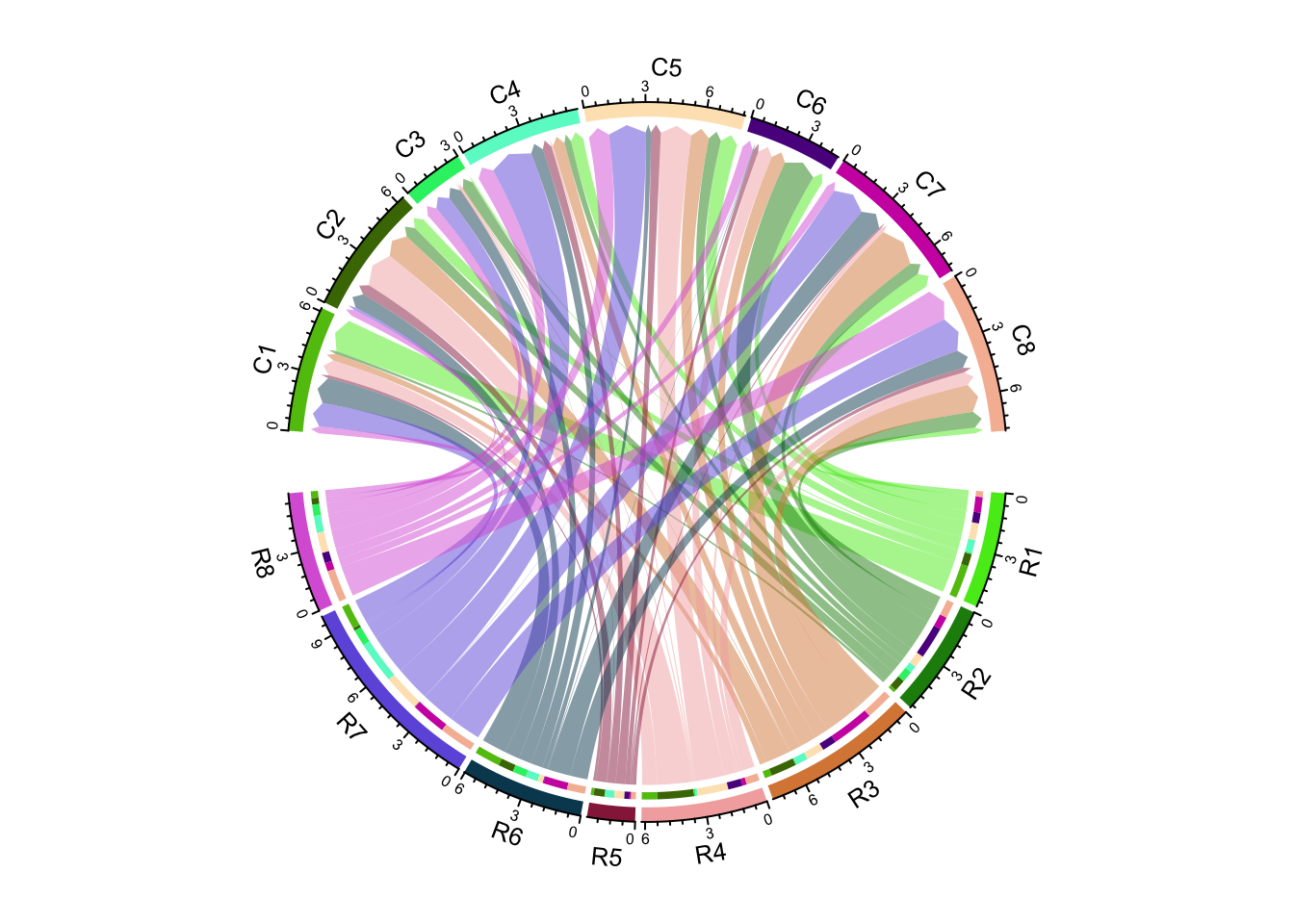Figure 14.28: Use big arrows to represent directions in Chord diagram.

If diffHeight is set to a negative value, the start ends are longer than the other ends (Figure 14.29).

chordDiagram(matx, directional = 1, direction.type = c("diffHeight", "arrows"),
link.arr.type = "big.arrow", diffHeight = -mm_h(2))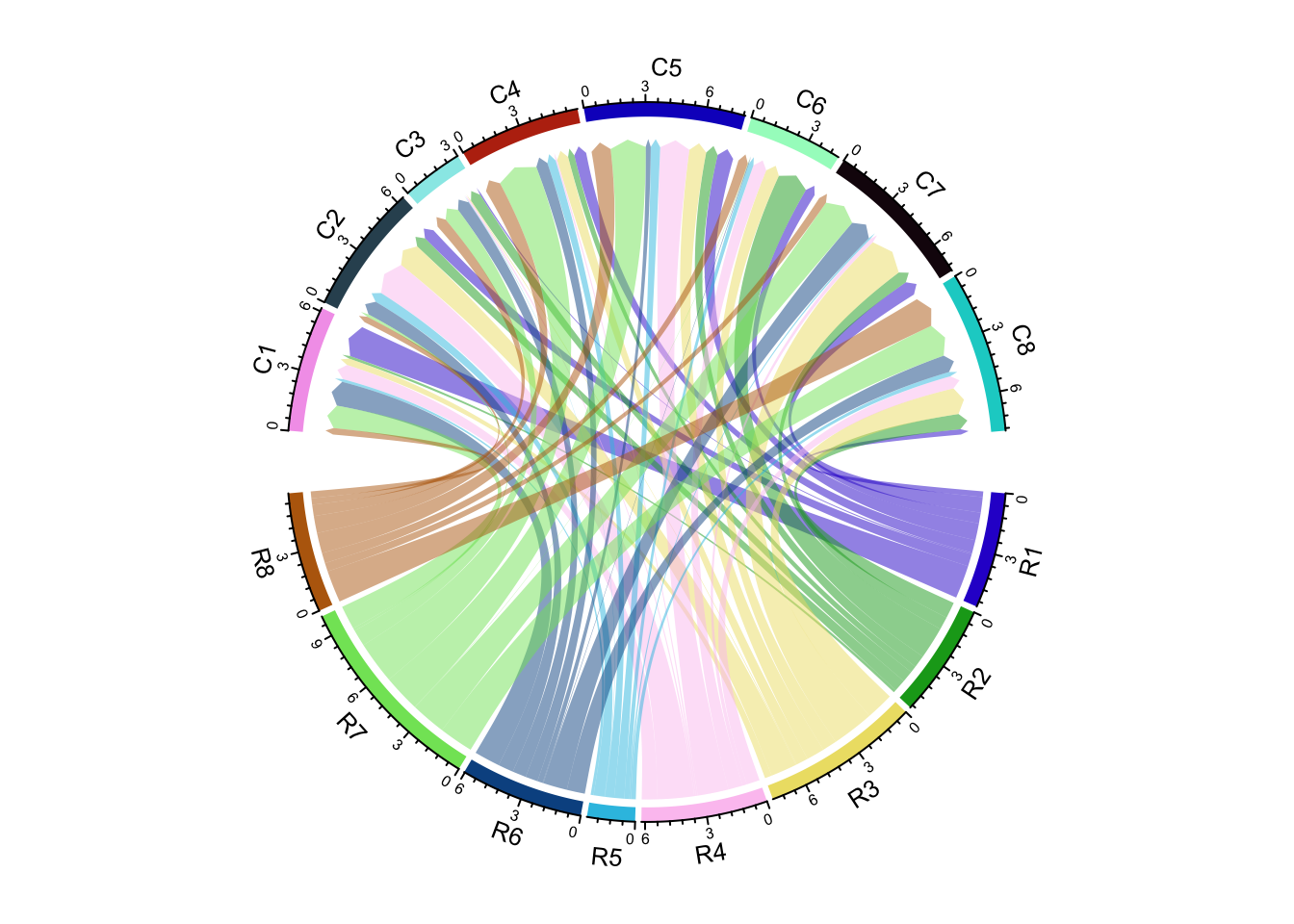Figure 14.29: Use big arrows to represent directions in Chord diagram.

It is almost the same to visualize directional Chord diagram form a adjacency list.

chordDiagram(df, directional = 1)

As shown in the previous Figures, there are bars on the source sector side that show the proportion of target sectors (actually it is not necessarily to be that, the bars are put on the side where the links are shorter and diffHeight with a positive value). The bars can be removed by setting link.target.prop = FALSE. The height of the bars is controlled by target.prop.height argument.

par(mfrow = c(1, 2))
chordDiagram(mat, grid.col = grid.col, directional = 1,
chordDiagram(mat, grid.col = grid.col, directional = 1,
diffHeight = mm_h(10), target.prop.height = mm_h(8))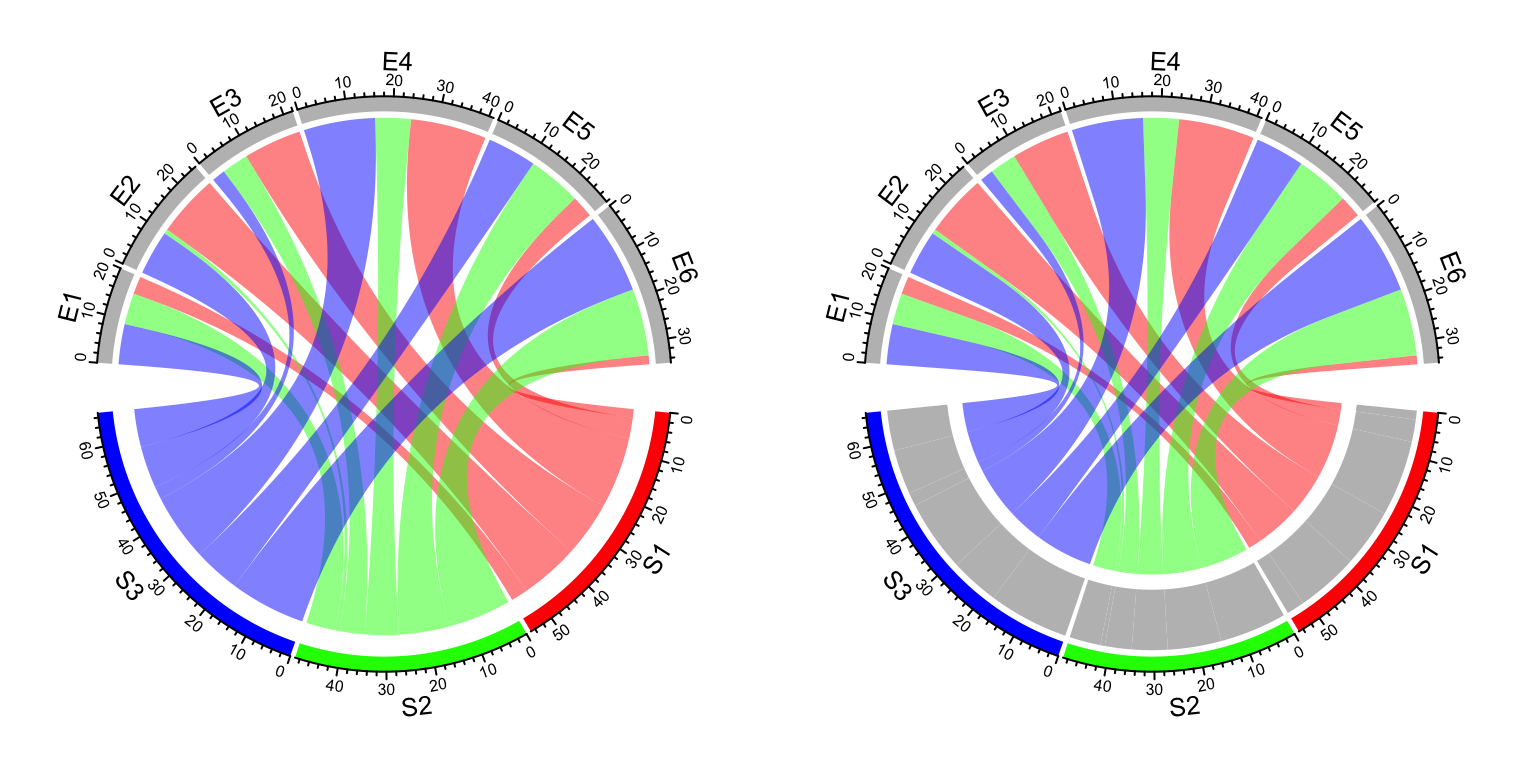Figure 14.30: Control argument link.target.prop and target.prop.height.

## 14.11 Scaling

The link on Chord diagram represents the “absolute value” of the relations. Sometimes for a certain sector, we might want to see relatively, how much of it goes to other sectors. In this case, scale argument can be set to TRUE so that on each sector, the value represents the fraction of the interaction going to one other sector (Figure 14.31).

set.seed(999)
mat = matrix(sample(18, 18), 3, 6)
rownames(mat) = paste0("S", 1:3)
colnames(mat) = paste0("E", 1:6)

grid.col = c(S1 = "red", S2 = "green", S3 = "blue",
E1 = "grey", E2 = "grey", E3 = "grey", E4 = "grey", E5 = "grey", E6 = "grey")
par(mfrow = c(1, 2))
chordDiagram(mat, grid.col = grid.col)
title("Default")
chordDiagram(mat, grid.col = grid.col, scale = TRUE)
title("scale = TRUE")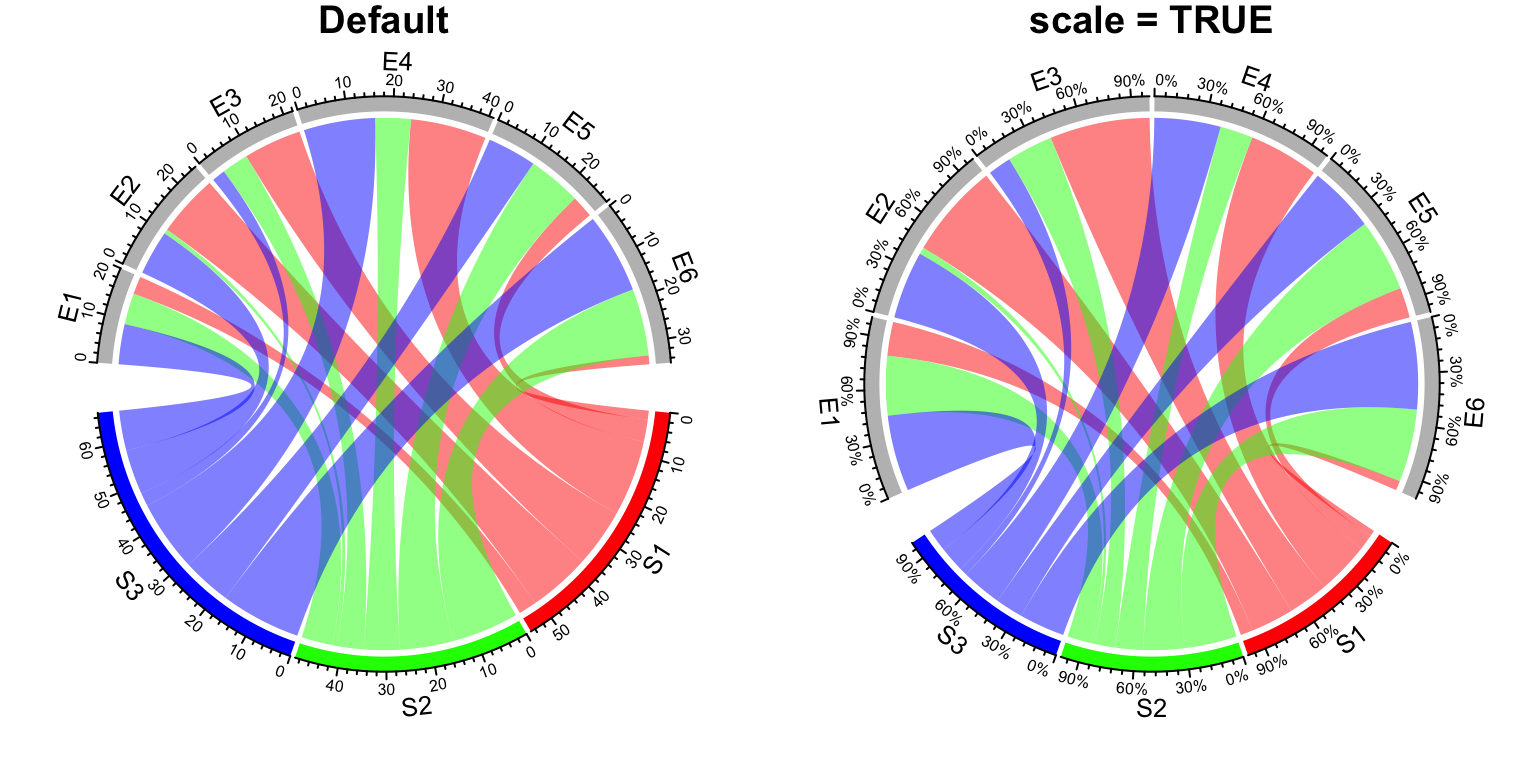Figure 14.31: Scaling on sectors.

After scaling, all sectors have the same size and the data range of each sector are all c(0, 1).

## 14.12 Reduce

If a sector in Chord Diagram is too small, it will be removed from the original matrix, since basically it can be ignored visually from the plot. In the following matrix, the second row and third column contain tiny values.

mat = matrix(rnorm(36), 6, 6)
rownames(mat) = paste0("R", 1:6)
colnames(mat) = paste0("C", 1:6)
mat[2, ] = 1e-10
mat[, 3] = 1e-10

In the Chord Diagram, categories corresponding to the second row and the third column will be removed (R2 and C3 are removed).

chordDiagram(mat)
circos.info()
## All your sectors:
##   "R1" "R3" "R4" "R5" "R6" "C1" "C2" "C4" "C5" "C6"
##
##  1 2
##
## Your current sector.index is C6
## Your current track.index is 2

The reduce argument controls the size of sectors to be removed. The value is a percent of the size of a sector to the total size of all sectors.

You can also explictly remove sectors by assigning corresponding values to 0.

mat[2, ] = 0
mat[, 3] = 0

All parameters for sectors such as colors or gaps between sectors are also automatically reduced accordingly by the function.

## 14.13 Input as a data frame

As mentioned before, both matrix and data frame can be used to represent relations between two sets of features. In previous examples, we mainly demonstrate the use of chordDiagram() with matrix as input. Also we show the code with data frame as input for each functionality. Here we will go through these functionality again with data frames and we also show some unique features which is only usable for data frames.

When the input is a data frame, number of rows in the data frame corresponds to the number of links on the Chord diagram. Many arguments can be set as a vector which have the same length as the number of rows of the input data frame. They are:

• transparency
• col. Note, col can also be a color mapping function generated from colorRamp2().
• link.border
• link.lwd
• link.lty
• link.arr.length
• link.arr.width
• link.arr.type
• link.arr.lwd
• link.arr.lty
• link.arr.col
• link.visible
• link.zindex

Since all of them are already demonstrated in previous sections, we won’t show them again here.

The following two sections list unique features only from data frames.